Negatory. Postpone Mission.

# npm

Miss any of our Open RFC calls?Watch the recordings here! »

## ball-morphology

0.1.0 • Public • Published

# ball-morphology

Mathematical morphology for ndarrays where the structuring element is a ball in some Lp metric.

# Example

Which gives us the following array: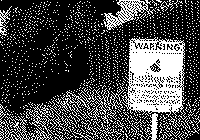We can dilate the image using the following command:

Which produces the following result: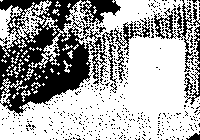Similarly, we can also perform an erosion:

Giving the result: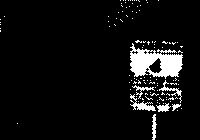For convenience, openings and closing are also implemented: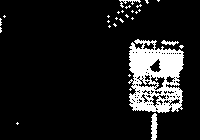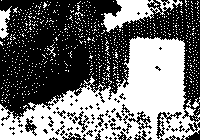## Install

``````npm install ball-morphology
``````

## API

### `morphology.dilate(array, radius[, p])`

Performs a binary morphological dilation with an Lp ball of a given radius

• `array` is a binary image (updated in place)
• `radius` is the radius of the ball in pixel units (may be fractional)
• `p` is an optional argument giving the exponent of the metric. (Default 2)

Returns `array`

### `morphology.erode(array, radius[, p])`

Performs a binary morphological erosion with an Lp ball of a given radius

• `array` is a binary image (updated in place)
• `radius` is the radius of the ball in pixel units (may be fractional)
• `p` is an optional argument giving the exponent of the metric. (Default 2)

Returns `array`

### `morphology.open(array, radius[, p])`

Performs a binary morphological opening with an Lp ball of a given radius

• `array` is a binary image (updated in place)
• `radius` is the radius of the ball in pixel units (may be fractional)
• `p` is an optional argument giving the exponent of the metric. (Default 2)

Returns `array`

### `morphology.close(array, radius[, p])`

Performs a binary morphological closing with an Lp ball of a given radius

• `array` is a binary image (updated in place)
• `radius` is the radius of the ball in pixel units (may be fractional)
• `p` is an optional argument giving the exponent of the metric. (Default 2)

Returns `array`

# Credits

(c) 2013 Mikola Lysenko. MIT License

## Keywords

none

### Install

`npm i ball-morphology`

4

0.1.0

none

### Repository

github.com/mikolalysenko/ball-morphology